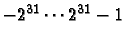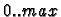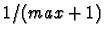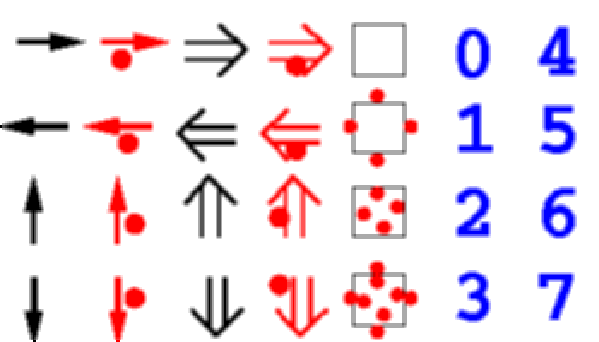Next: 4. Efficient simulation of Up: 3. Languages for Cellular Previous: 3.1 Specification of CA   Contents

Subsections

# 3.2 CDL

The language CDL (Cellular Description Language) was developed by Chistian Hochberger and his group in Darmstadt  to describe cellular automata for simulation on special purpose hardware (the CEPRA family of machines) and in software. The main idea was to create a language that supports those features which are necessary for simulating CA, but is not a general-purpose language,and thus hopefully simpler to use. Also, special features of cellular automata, such as access to the neighboring cells and operations over all neighbors are supported by simple constructs. Here we use a dialect of CDL extended by a few new features. In the CASim system, descriptions of cellular automata in the CDL language are translated automatically into Java code, which is then compiled and loaded. One drawback of this two-step process is that some programming errors (e.g., uninitialized variables) are only found during the compilation of the Java code, and the user has to trace the error back to the corresponding CDL code. The advantage is that the user can continue using the Java code instead of the CDL code, thus taking advantage of the more general capabilities fo Java.

## 3.2.1 CDL Example

A simple example of a cellular automaton is shown below. The program is explained in the following section.

```
cellular automaton GH5;

const maxvalue = 4;
type celltype = 0 .. maxvalue;

color [0, (255 * *[0, 0])/maxvalue, 0] ~ *[0, 0]>0;
initial maxvalue ~ prob(0.03);

group neighbors = {*[0,1],*[0,-1],*[1,0],*[-1,0]};
var   xx :  celltype;
const constantcell = ;

rule  begin
if *[0, 0] > 0 then
*[0, 0] := *[0, 0] - 1
else
if one(xx in neighbors : xx = maxvalue) then
*[0, 0] := maxvalue
else
*[0, 0] := 0;
end;
```

## 3.2.2 CDL Basics

The language CDL uses a syntax similar to PASCAL. In principle, a CDL-program describes one cell and its transition function. Additional code can be used to describe the visualization of a cell and the initial conditions. A CDL program consists of a number of sections, each of which is introduced by a section keyword. Sections can be repeated and mixed in any order (except for the rule sections, which must follow all other sections). CDL is case sensitive, but all keywords exist in two versions, on all lower case and one all upper case. The list of reserved words is:

```
abs     all       and      automaton begin  boolean
border  case      cellular colortype color  const
default div       do       element   else   end
false   float     for      function  global group
hsv     if        in       initial   int    integer
mod     next      not      num       of     one
or      otherwise prev     prob      random record
return  round     rule     sum       switch then
true    trunc     type     union     value  var
xor
```
Of these, the keywords `initial`, `prob`, and `sum` are newly introduced here and did not appear in the original CDL definition.

In addition to the reserved keywords, there are a number of reserved identifiers, which are used for special purposes and should not be used for other purposes:

```
celladdress  celltype  cell dimension  distance
length   lx  ly  lz    option    x  y  z
constantcell
constantcellleft constantcellright
constantcelltop constantcellbottom
constantcellfront constantcellaft
```

The name-space for identifiers is flat, which means that each identifier may only be used once, so an identifier that is used as the name for a rule may not appear as a component of a type or as an element in an enumeration.

The meaning and usage of the keywords and special identifiers is explained in the following sections. The complete grammar is described in the appendix. A cellular automaton description starts with the keywords

```
cellular automaton
```
followed by a name and a semicolon. For the translation in the CASim system, the name must be identical to the filename (without the `.cdl` ending).

## 3.2.3 Type declaration

The first key section is type for the declaration of types. This section contains type declarations of the form

name = type;
where name must be an identifier which has not yet been declared, and type is one of the types described below. The special type celltype must be declared in every program, since it describes the data stored in each cell and there is no default value.

The basic data types available are:

• `integer`: an integer (which is theoretically unlimited and therefore should only be used when the restriction of cellular automata to finite state sets is not relevant). In the CASim system, an integer has the same definition as a JAVA integer, i.e., a range of.

• `float`: a floating point variable (which is theoretically unlimited and therefore should only be used when the restriction of cellular automata to finite state sets is not relevant).

• `boolean`: a boolean value, which can take the values of `true` or `false` (both are predefined constants) and can be used in logical expressions and in `if`-statements.

• An enumeration, which is written as
`(value1, value2, value3)` for the case of an enumeration of three values. Note that each enumeration value may only appear once due to the flat name-space.

• A range is a subtype of an integer with a minimum and maximum value given explicitly. The syntax for this type is `min .. max` and it is translated into an integer type in the CASim system.

These basic data types can be combined to composite type with the `record` or `union` keywords. Records consist of a number of components and are declared as in the following example:

```
type celltype = record
mycolorname: (red, blue, green);
excited: boolean;
age: 0..100;
end;
```

The components of a variable (say `a`) of this type are then accessed using the dot as in `a.mycolorname` or `a.age`. A component of a record may also be a record. The CDL-language also provides the concept of `union` with the same syntax as for the record, but with the semantics that the hardware or compiler may store all components in the same storage space.

## 3.2.4 Variables

The second key section is var for the declaration of temporary variables. These variables may be used inside the `rule`, but do not keep their values from one generation to the next, i.e., they are not part of the cell state.

This section contains variable declarations of the form

var name : type;
name1, name2 : type;

where name is a new identifier or a list of identifiers as in name1, name2, and type is a basic data type, a named type declared in the `type` section, or a compound type as used in the declaration of a type.

## 3.2.5 Transition Rule

Normally, there is only one `rule` section in a CDL program. This rule describes the state transition function. The `rule` section must succeed all other sections. A rule my be named, but currently only the special rule `rule global` is supported in combination with the `global` section described below.

In the rule section, CDL follows an imperative paradigm. The rule consists of a number of statements, of which the assignemnt statement is the most important. The possible statements are:

Assigment:
lhs := rhs ;

If:
if expression then statement ;
if expression then statement1 else statement2 ;

Block:
begin statement1 ; statement2 ; ... end

Additional statements are the `case` statement and the `for` statement, which are described below.

The assigment assigns a new value to the left hand side from the right hand side expression. The left hand side may either be a variable, in which case the asignment has the usual semantics known from other imperative programming languages. If the lhs is a reference to the cell or a component of the cell state, then the assigmnent means that at the end of the updating step, this component of the cell gets the new value. The change does not happen immediately, since the semantics of cellular automata require that references to a cell or a neighbor always refer to the previous time step to make sure that the updating is really fully synchronous. Therefore a reference to a cell on the RHS of an assignment or in any other expression always refers to the old state.

The `if`-statement has the usual semantics. Note that if more than one statement needs to be included in the `if`-statement, a block with `begin .. end` must be used. Also note that there is no semicolon after the first statement in the `if then else` statement.

## 3.2.6 Expressions

In CDL, values can be combined using expressions as in most other programming languages. The available operators are:
```
+  -  *  /  div  mod  and  or  not  xor
=  !=  <  >  <=  >=
```
Most of these operators should be self-evident. The operator `div` is an integer division, while `/` results in a float. The logical operators operate on boolean values or expressions only, and do not provide bitwise operations on integers. Comparisons for equality or non-equality may be applied to compound data types (such as records), all other operations may only be applied to basic data types. Normal parentheses are available for grouping expressions and ovveriding the default precedence rules (which can be inferred from the grammar).

A new conditional operator `` expr ? value1 : value2'' has been introduced since it is present in C and Java and makes coding of some constructs easier.

The reference to the state of the cell or the state of a neighboring cell is achieved through the use of a dereference operator, which operates on a celladdress. A celladdress usually is a constant record with as many integer components as the cellular automaton has dimensions. A celladdress can also be a variable or even a record component of type `celladdress`. The type `celladdress` is an implicitly defined record with components `x,y,z` (using the constants `dimension` and `distance` if they are defined or can be inferred).

A normal reference to a cell in a two-dimensional automaton is `*[0,0]`, while `*[1,0]` refers to the right neighbor. Using the compound celltype given above as an example, one can write statements like

```
*[0,0].age := ( *[0,1].age + *[0,-1].age ) div 2;

if *[1,0].excited and *[0,0].age > 0 then
*[0,0].age := *[0,0].age - 1;
```
If a variable of type `celladdress` is defined, e.g., by
```
var n: celladdress;
```
then this variable can be used:
```
n := [0,1];
*n.mycolorname := blue;
```

There are a number of special operations which use ``groups'' and these are described in the following section.

## 3.2.7 Groups

In CDL a concept of ``groups'' is introduced. Groups a basically lists of constant elements of the same type. They are used in special group operations, namely the `for`-statement and the functions `one`, `all`, `num`, and `sum`. The last of these (`sum`) is newly introduced in this version of CDL. The way groups are use in these constructs is that a variable is assigned each element in a group in sequence, and some evaluation is done or a stament is executed.

A group is declared in a special group section:

group groupname = { element1, element2, .. };

These groups are then used in a `for`-statement or in special group functions. The `for`-statement has the following syntax:

for variable in groupname do
statement ;

It is also possible to use multiple variables and groups as in:

for variable1 in groupname1 & variable2 in groupname2 do
statement ;

In this case the system does not produce a nested loop, but rather in the first iteration assigns the first element of groupname1 to variable1 and the first element of groupname2 to variable2. In the second iteration the second elements of the respective groups are used.

In the original version of CDL another possibility is defined, where multiple variables use the same group, and are assigned consequetive elements, but this usage is discouraged and not fully supported in CASim.

The functions `one`, `all`, `num`, and `sum` similarly loop over all elements of the group and perform some evaluation of an expression.

one( variable in groupname : expression )

The result of the function `one` is true if the expression (which should use the variable) is true for at least one iteration. Similarly, `all` is true if the expression is true for all iterations, and `num` counts the number of iterations for which expression is true. The function `sum` calculates the sum of expression over all iterations. In this case expression should be of type integer or float.

## 3.2.8 Functions

The original definition of CDL includes user-defined functions. These are not yet supported in CASim. A number of predefined functions are provided:
• random() The function random( max ) returns a random integer number in the range, where both endpoints are included and each value has equal probability.
• prob() The function prob( p ) returns a boolean value which is true with probability p.
• value() The function value( enumvalue ) is used to obtain an integer representation for an element of an enumeration.
• next() The function next( enumvalue ) returns the successor of an element of an enumeration. For the last element, periodic continuation is used, and the first element returned.
• prev() The function prev( enumvalue ) returns the predecessor of an element of an enumeration. For the first element, periodic continuation is used and the last element returned.

## 3.2.9 Constants

The keyword const introduced a section of constant declarations. These can be used to make a CDL-description more easily readable. Each constant is declared by
const name = value;

where value may be a complex expression. The example
```
const
maxvalue = 4;
c = *[0,0];
blue = [0,0,255];
```
declares an integer constant maxvalue, a contant c which can be used to refer to the current cell, and a color blue, which can be used in the color section.

The special constants `dimension` and `distance` are used to determine the properties of the type `celladdress`. `dimension` should have a value between 1 and 3, and `distance` a value that gives the maximal extent of the neighborhood in any direction. In many cases the values of these constants can be inferred automatically, and this is done in the CASim system. The original CDL requires explicit definition of these constants in any case.

Another set of special constants may be defined in the CASim system to specify constant boundary values:

```
constantcell
constantcellleft constantcellright
constantcelltop constantcellbottom
constantcellfront constantcellaft
```

These constants are used by the simulation system if the user selects constant boundary conditions (as opposed to periodic of reflective boundaries). The constant `constantcell` is used for all boundaries which do not have a different value declared explicitly.

## 3.2.10 Color and Icons

In the simulation system CASim, cellular automata can be visualized using one of three options. Cells can be represented by text, colored fields, or icons. The translation system automatically produces code to prepare a textual representation of each cell using the usual printed representation of the data types of which the cell state is composed.

The second simplest option is to define colors for the representation of the cell. In the CDL code, this is done in the `color` section, which contains color specifications of the form
color [ reb, green, blue ]   condition;

where condition is a boolean expression which may involve references to the cell state (e.g., `*[0,0]`) and constants, but not to the neighbor states or to variables. conditions are evaluated from top down, and the first condition which is true determines the color. Thus a default color can be specified by adding `[r,g,b] ~ true` at the end of all other color specifications. If no condition evaluates to true, the cell will be transparent in CASim.

The color specification is a triplet of RGB-values in the range 0..255. To aid in the construction of colors, a special function `hsv` is provided, which takes three arguments as hue, saturation, and brightness (in the range 0..1) and converts them into RGB values. Examples of color specifications are:

```
color [255* *[0,0].a , 0, 0]  ~ *[0,0].b = 0;
hsv( *[0,0].a,1,1)      ~ *[0,0].b = 1;
hsv( 0,1,*[0,0].a)      ~ *[0,0].b = 2;
[0,0,0]                 ~ true;
```
Note that the cell state may be used in the calculation of the RGB values as well as in the condition.

A third possibility is to use icons to represent cell states. Icons can not easily be parameterized, but instead one icon must represent one or several state. In the simulation system CASim support is provided for images that contain a number of icons. One instruction can then select an icon from the image based on the cell state. The syntax of this statement is in cluded in the color section, where instead of a triplet of RGB values, an icon is specified by an image filename and a selector of one icon from this file of the form

`` filename.gif'' ( ix: ilx, iy: ily)

where the image file may be a GIF image or a JPEG image. The selector of the form ( ix: ilx, iy: ily) must consist of constant numbers ilx and ily which indicate the number of icons present in the image in x- and y-direction, and of two expressions ix and iy which indiate the icon to select from the ilx* ily field of icons. The expressions ix and iy may contain references to the cell state. An example file is shown in Figure 3.1, and the code selecting icons from this image is

```
color
"arrvn.gif" ((*c.excited?1:0)+(value(*c.typ)-1)*2 :7, 0:4)
~ *c.typ in {normt, spect} and *c.dir = east;
"arrvn.gif" ((*c.excited?1:0)+(value(*c.typ)-1)*2 :7, 1:4)
~ *c.typ in {normt, spect} and *c.dir = west;
"arrvn.gif" (4:7, value(*c.attribut.cstate):4)
~ *c.typ = conf;
"arrvn.gif" (5 + (value(*c.attribut.sstate) div 4):7,
(value(*c.attribut.sstate) mod 4):4)
~ *c.typ = sens;
```
where the first line is used to select one of four icons in the first row and first four columns, the second line for the corresponding icons in the second row, and the last expression selects one of four icons in the fifth column and the last expression selects one of the eight numbers from the right. Color and icon selectors may be mixed in the CDL code. The order is important, since the first matching expression determines the appearance of a cell. The image file must be located in the same directory as the CDL source code. It is read at the time of translation from CDL to Java, and is included in the Java code. Therefore the image file need not be transported to a presentation directory if a WWW-presentation is prepared with the .class files.## 3.2.11 Initial conditions

The creators of CDL decided to separate initial conditions from the description of the CA transition rule with the argument that the user would want to do many experiments with different initial conditions but the same transition rule. In the CASim system, we have decided to include initial conditions in the description of the CA, and provided a simple mechanism for doing so in CDL. The `initial` section is introduced by the keyword `initial` and consists of pairs of records and expressions similar to the `color`-section. The records must contain one element for each component of the cell state, regardless of the hierarchy level. The expressions may use the special symbols x, y, z which give the position of the cell in the lattice of size lx, ly, lz. The conditions may also use the special variable option, which can be set to an integer value from the simulation system, e.g., to distinguish between different possible experiments.

If the cell type is declared as

```
type celltype = record
typ : (unex, normt, spect, conf, sens);
excited :  boolean;
dir : celladdress;
attribut: record
cstate :(zz, zo, oz, oo);
sstate :(si, sz, so, szz, szo, soz, soo, szzz);
end;
end;
```
(the definition used for the von Neumann 29 state self reproducing automaton), then valid initializations are:
```
initial
[normt, false, 1, 0, zz, szz ]
~  x >= lx/2 and  y = ly-1 and option = 1 ;
[normt, true, 0, 1, zz, szz ]
~  x = lx/2 and  y = ly/2 and option = 1 ;
[normt, false, 0, 1, zz, szz ]
~  x = lx/2 and  y > ly/2 and option = 1 ;
[spect, prob(0.2), 1, 0, zz, szz ]
~  option = 0 and prob(0.2);
[unex, false, 0, 0, zz, szz] ~ true;
```
are valid initializations.Next: 4. Efficient simulation of Up: 3. Languages for Cellular Previous: 3.1 Specification of CA   Contents
Jörg R. Weimar